1
2
3
4
5
#

Correlation

I. Introduction
When analyzing bivariate data that has a linear relationship, correlation, also know as the correlation coefficient and commonly referred to simply as "r", is used to measure both the:

1. strength and
2. direction of a linear relationship.

II. Correlation Examples

 EXAMPLE SCATTERPLOTS WITH CORRELATION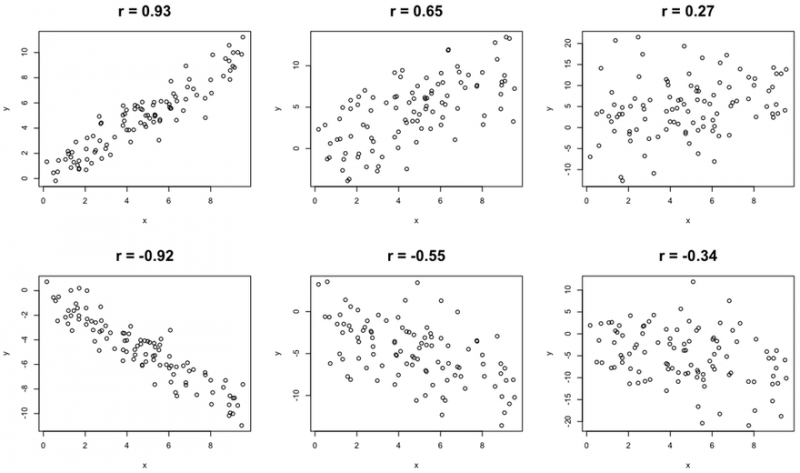III. Correlation Facts

(1) Correlation is a measure of the linear (straight-line) relationship between two variables.

(2) Correlation is calculated using the formula below. It is important to note, however, that correlation is virtually never calculated by hand, and almost always is calculated using technology, such as a statistics enabled calculator or statistics package.

Correlation Formula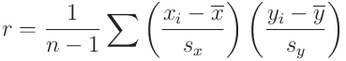(3) If r is zero or approximately zero, there can be two possible reasons.

 Reason 1: There is weak linear relationship between the variables. Reason 2: There is a strong relationship between the two variables, but the strong relationship may be nonlinear.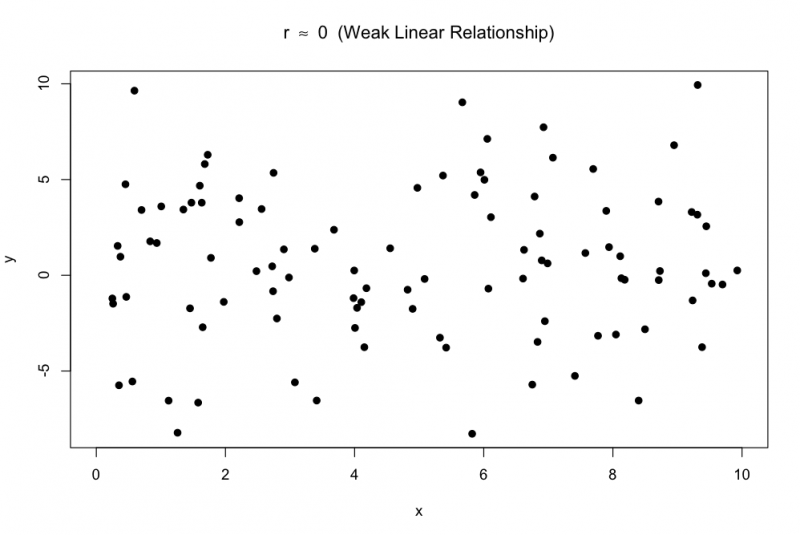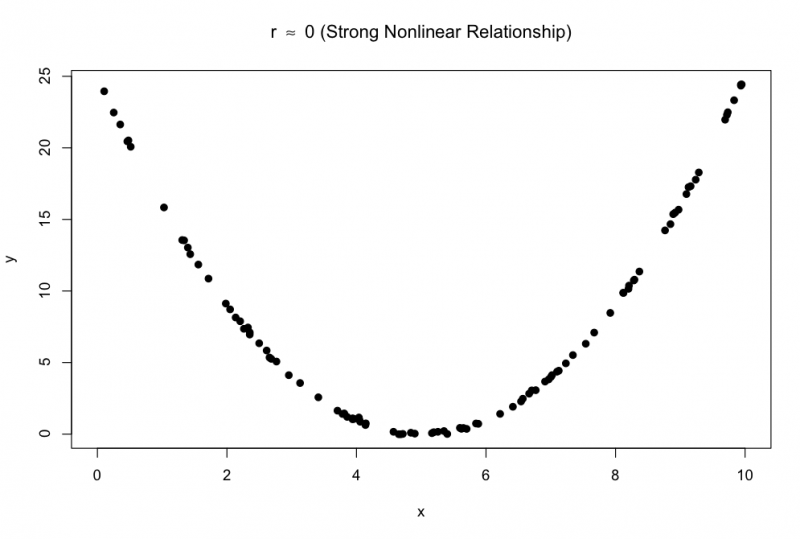(4) Correlation has a range from -1.00 to +1.00 and the sign (positive or negative) of the correlation coeﬃcient indicates the direction (positive or negative) of the linear relationship between X and Y.

(5) If correlation equals -1 or +1:

 If r = +1, we say X and Y are perfectly positively correlated, and all of the points would form a straight line. If r = −1, we say X and Y are perfectly negatively correlated, and all of the points would form a straight line.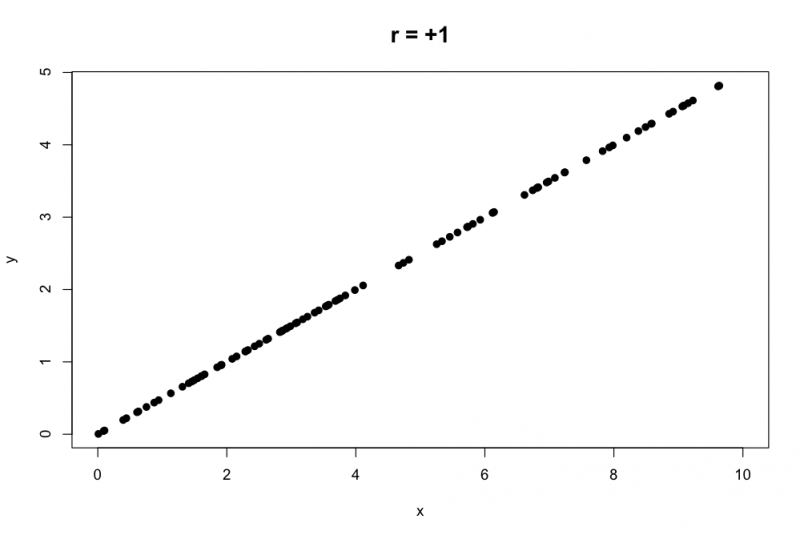(6) The magnitude (absolute value, distance from zero) of the correlation coeﬃcient indicates the strength of the
linear relationship between X and Y.

(7) The correlation coeﬃcient (like the mean and standard deviation) can be greatly aﬀected by outliers.

(8) CORRELATION IS NOT CAUSATION!!! Just because X and Y are correlated, it does not mean
that one causes the other.